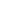#Learning Excel: Data-Analysis (LinkedIn Learning) is a Course

## Learning Excel: Data-Analysis (LinkedIn Learning)

Started May 1, 2020

### Full course description

Term: Spring 2020
Date, Time & Location: Online Self-Paced Course
Instructor: Curt Frye
Curated By: Talent Development via LinkedIn Learning

Description:

Learning Excel: Data Analysis (LinkedIn Learning) is an important tool that helps users accurately assess situations and make better business decisions. This course helps you unlock the power of Virginia Tech’s robust data using the data analysis and visualization tools built into Excel. Author Curt Frye starts with the foundational concepts, including basic calculations such as mean, median, and standard deviation, and provides an introduction to the central limit theorem. He then shows how to visualize data, relationships, and future results with Excel's histograms, graphs, and charts. He also covers testing hypotheses, modeling different data distributions, and calculating the covariance and correlation between data sets. Finally, he reviews the process of calculating Bayesian probabilities in Excel. Each chapter includes practical examples that show how to apply the techniques to real-world business problems.

### Learning Objectives:

• Calculating mean and median values
• Analyzing data using variance and standard deviation
• Working with sample data
• Minimizing errors
• Visualizing data with histograms, charts, and more
• Testing hypotheses
• Measuring covariance and correlation
• Performing Bayesian analysis